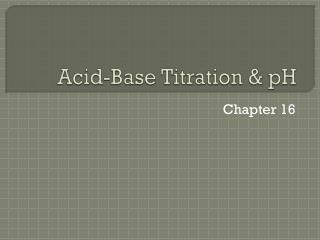DownloadDownload PresentationAcid-Base Titration & pH

# Acid-Base Titration & pH

Télécharger la présentation## Acid-Base Titration & pH

- - - - - - - - - - - - - - - - - - - - - - - - - - - E N D - - - - - - - - - - - - - - - - - - - - - - - - - - -
##### Presentation Transcript

1. Acid-Base Titration & pH Chapter 16

2. Neutral, Acidic & Basic Solutions • [H3O+]=[OH-] (solution is neutral) • Occurs in pure water • Acids increase concentration of H3O+ • Bases increase concentration of OH-

3. pouvoirhydrogène (Fr.) “hydrogen power” pH Scale 14 0 7 INCREASING BASICITY INCREASING ACIDITY NEUTRAL pH = -log[H3O+]

4. pH Scale pH = -log[H3O+] pOH = -log[OH-] pH + pOH = 14

5. pH Scale • What is the pH of 0.050 M HNO3? pH = -log[H3O+] pH = -log[0.050] pH = 1.3 Acidic or basic? Acidic

6. pH Scale • What is the molarity of HBr in a solution that has a pOH of 9.6? pH + pOH = 14 pH + 9.6 = 14 pH = 4.4 pH = -log[H3O+] 4.4 = -log[H3O+] -4.4 = log[H3O+] [H3O+] = 4.0  10-5 M HBr Acidic

7. Titration Determining an unknown

8. standard solution unknown solution Titration • Titration • Analytical method in which a standard solution is used to determine the concentration of an unknown solution.

9. Titration • Equivalence point (endpoint) • Point at which equal amounts of H3O+ and OH- have been added. • Determined by… • indicator color change

10. Titration moles H3O+ = moles OH- MVn = MVn M: Molarity V: volume n: # of H+ ions in the acid or OH- ions in the base

11. Titration • 42.5 mL of 1.3M KOH are required to neutralize 50.0 mL of H2SO4. Find the molarity of H2SO4. H3O+ M = ? V = 50.0 mL n = 2 OH- M = 1.3M V = 42.5 mL n = 1 MV# = MV# M(50.0mL)(2) =(1.3M)(42.5mL)(1) M = 0.55M H2SO4